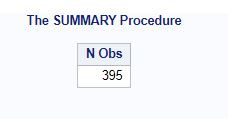Search
•Ekta Aggarwal

# PROC SUMMARY vs PROC MEANS

PROC SUMMARY has same utility as PROC MEANS which one of the most powerful procedures in SAS used to calculate various summary statistics like mean, median, count, count of missing values, standard deviation, range, percentiles and many more for NUMERIC columns in our data.

In this tutorial we shall try to understand the differences between PROC MEANS and PROC SUMMARY.

For this we shall make use of inbuilt SAS dataset SASHELP.SHOES

### Mentioning PRINT KEYWORD is mandatory in PROC SUMMARY.

Skipping PRINT keyword in PROC SUMMARY would lead to errors while PROC MEAN by default prints the output

```PROC SUMMARY DATA = SASHELP.SHOES;
RUN;```## Skipping VAR statement in PROC SUMMARY

If you skip VAR statement in PROC SUMMARY then it will result in number of rows as the output. Although skipping the VAR statement in PROC MEANS calculate summary statistics for all the numeric columns.

```PROC SUMMARY DATA = SASHELP.SHOES PRINT;
RUN;```Thus to mention the VAR statement in PROC SUMMARY is a must, then only it will provide similar output as PROC MEANS.

```PROC SUMMARY DATA = SASHELP.SHOES PRINT;
VAR SALES RETURNS INVENTORY;
RUN;```Just like PROC MEANS you can define summary statistics and CLASS statements in PROC SUMMARY as well.

```PROC SUMMARY DATA = SASHELP.SHOES PRINT MEAN MEDIAN RANGE;
VAR SALES RETURNS INVENTORY;
RUN;```